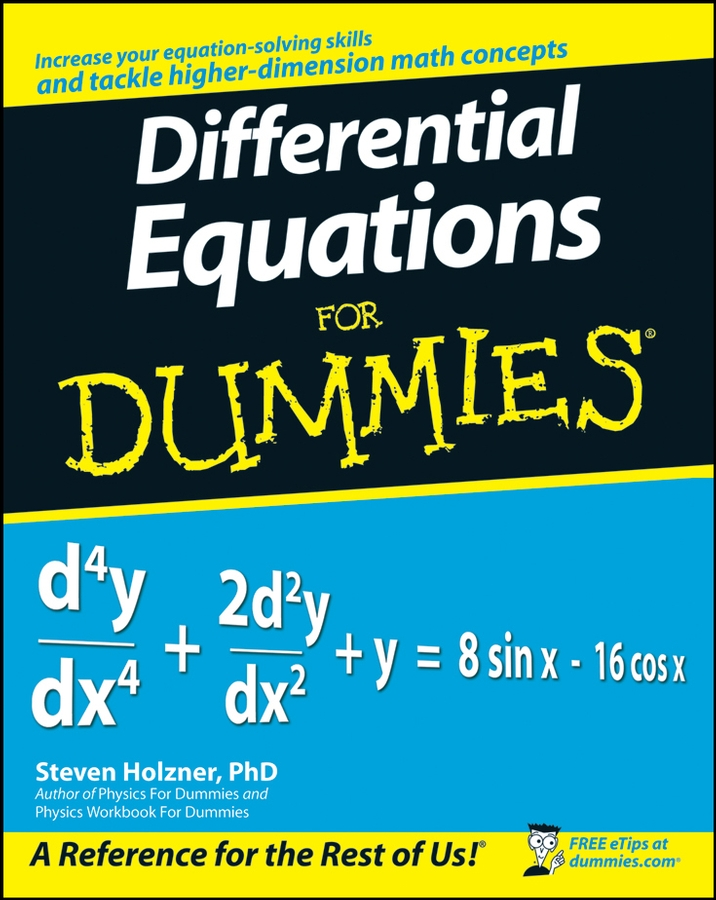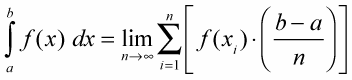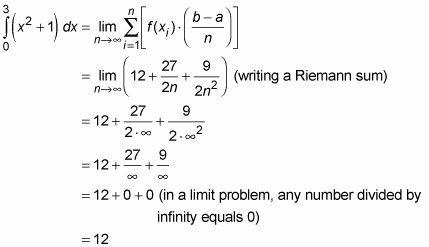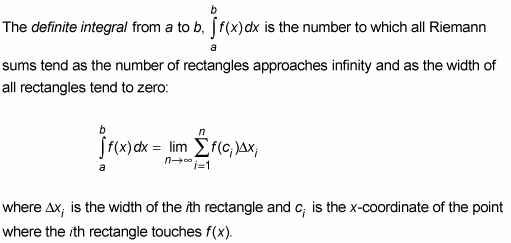##### Differential Equations For DummiesYou can approximate the area under a curve by adding up right, left, or midpoint rectangles. To find an exact area, you need to use a definite integral.

When you approximate the area under a curve, the tops of the rectangles form a saw tooth shape that doesn’t fit perfectly along the smooth curving function. So, to find the exact area under a curve using rectangles, you’d need to find the area of an infinite number of infinitely thin rectangles whose “tops” do perfectly fit the curve. Now, you can’t really use an infinite number of rectangles, but with the fantastic invention of limits, this is sort of what happens.

Here’s the “simple” definition of the definite integral that’s used to compute exact areas. It’s based on the limit of a Riemann sum of right rectangles. The exact area under a curve between a and b is given by the definite integral, which is defined as follows:When calculating an approximate or exact area under a curve, all three sums — left, right, and midpoint — are called Riemann sums after the great German mathematician G. F. B. Riemann (1826–66). Basically, any sum made up of rectangles is a Riemann sum, including weird sums consisting of rectangles of unequal width.

Using this formula, here is the exact area under x2 + 1 between 0 and 3:This result is pretty amazing if you think about it. Using the limit process, you get an exact answer of 12 — which is sort of like 12.00000000… accurate to an infinite number of decimal places — for the area under the smooth, curving function, x2 + 1, based on the areas of flat-topped rectangles that run along the curve in a jagged, saw-tooth fashion.

Finding the exact area of 12 by using the limit of a Riemann sum is a lot of work. This complicated method of integration is comparable to determining a derivative the hard way by using the formal definition that’s based on the difference quotient. And like using the difference quotient to find a derivative, you won’t use the limit of a Riemann sum to calculate area once you learn the shortcut method of finding area.

You just worked out the area under x2 + 1, using the formula for the sum of right rectangles. (By the way, if you are going to compute area the hard way using the limit of a Riemann sum, you might as well always use right rectangles; it’s probably the easiest way to go). It turns out, though, that you could have used left rectangles or midpoint rectangles. In fact, you could have used a mix of different types of rectangles (don’t try this at home). No matter what type of rectangles you use, the limit always comes out the same.

This brings you to the following totally extreme, down-and-dirty integration mumbo jumbo that takes all these possibilities into account. You’ll probably never use the following definition of the definite integral. So why should you learn it? Good question. How about because of the beauty of the pure mathematics? Or, if you don’t buy that and are only interested in a practical reason, just about the only practical motivation for learning this definition is that it might be on an exam.

The Definite Integral (real-McCoy definition):